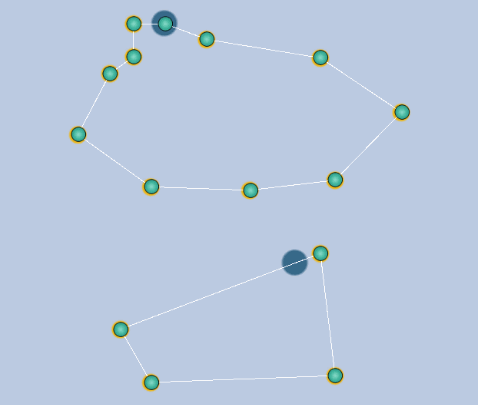# MoveTowards on paths, different speeds

Hello, Object is moving by MoveTowards(transform.position, points[index], speed). But sometimes on path I have a larger cluster of points, thus the object slows down. As you can see on picture, object a will be faster than object b, they are moving with the same *speed. What can I do to make object not slowing down where points will be more? I know why it slow down but I dont know what can I use instead movetowards. I tried with lookat and transform translate with vector3.forward but with faster speed object starts to lose because it jumps over more than one point during one frame.
[129378-szkic-2.png|129378]

I’m not sure for what purposes you are using curves but from the first impression I think you should be using interpolation instead of MoveTowards because interpolation will make sure the point is between A & B consider time is in [0-1] range.

``````transform.position = Vector3.Lerp( p1, p2, time );
``````

As you have figured it out there is a speed issue when it breaks on shorter paths etc. For this you need to set a consistent speed, imagine something like km/h.

``````float distance = ( p1 - p2 ).magnitude; // distance between current segment
speedFactor = ( 10.0f / distance ); // 10.0f is some custom factor
``````

Speed factor makes sure the speed is consistent through the path. On short paths it will be bigger while on longer ones it will be smaller.

With speed factor calculated we can properly set the time which you need to feed the Vector3.Lerp method.

``````time += Time.deltaTime * speedFactor * speed;

if( time >= 1.0f )
{
time -= 1.0f;
SetNextPath();
}

transform.position = Vector3.Lerp( p1, p2, time );
``````That’s rough explanation of solution to your problem. Here is a whole piece of code without the part where you get the next segment. I believe you have this figured out already.

``````public float moveSpeed = 1.0f;
private float speedFactor = 0.0f;
private Vector3 p1;
private Vector3 p2;

void Update() {
time += Time.deltaTime * speedFactor * speed;

if( time >= 1.0f )
{
time -= 1.0f;
SetNextPath();
}

transform.position = Vector3.Lerp( p1, p2, time );
}

// This method sets next segment between which object moves and calculates new speed factor
void SetNextPath() {
// get next segment of points (p1,p2)

float distance = ( p1 - p2 ).magnitude; // distance between current segment
speedFactor = ( 10.0f / distance ); // 10.0f is some custom factor
}
``````

Here you go @Wenon. I’m not expert and there might be other, better solutions to your issue like Bézier curves etc. but this should still work in any case. Let me know how it goes!